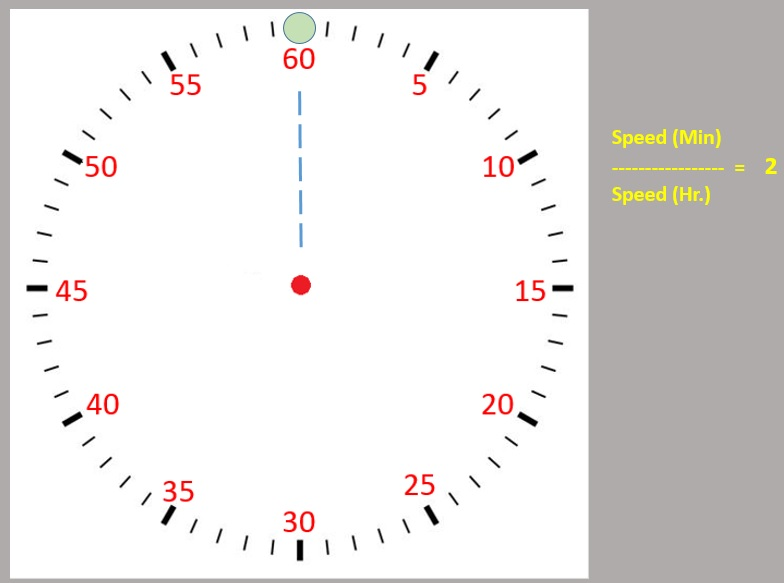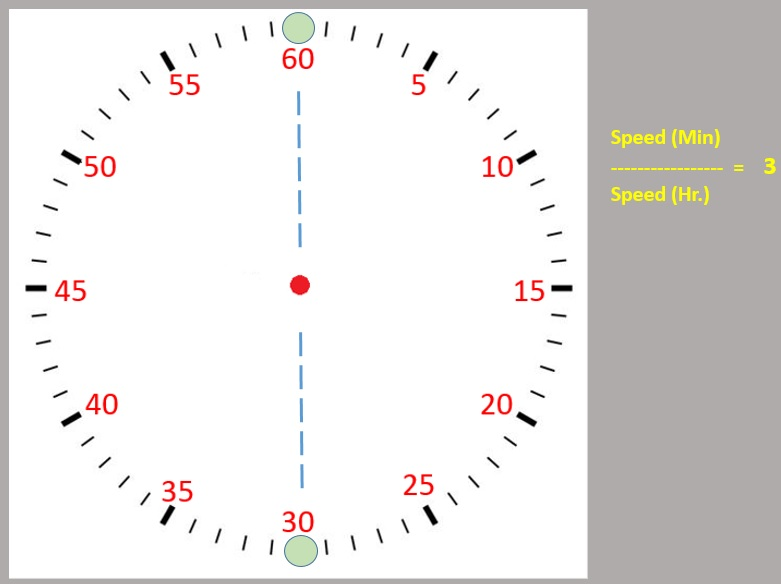# Analysis - Clock Hands Meet Puzzle & Generalization to N hands

Page 1 of 2

### "How many times do clock's hour and minute hands meet in a 12 hr. period? What is the time at the point where they meet?"

This is a problem everyone has heard and tried to solve at some point of time. Few days ago I overheard some teenagers having a conversation about this and the problem revolved in my head again. Though 'how many times' is a simple question to answer, the exact time when they meet is a little tricky. Looking at it as if the minute hand is chasing the hour hand, we run into sum of infinite series.Sometimes, the 2nd part of the question requires you to know the answer to the first part, which kind of feels like "cheating" to get the answer to the second one. :) I decided to give it some more thought and this is what I came across. Here, we also extend our solution to figure out a general case for a clock with N hands.

Before we start, assume that the clock's perimeter is divided into 60 ticks (which are minutes). We can also assume 360 ticks, one for each degree, but it does not matter. We will stick to 60 ticks. For all cases, we will assume that the initial position of the hands is at 12'0 clock before the hands start moving. We will stick to hands or points moving along a 2D plane.

In the conventional clock, the speed of the minute hand is 12 times the speed of hour hand. The hands meet 11 times in 12 hr period. 12 and 11, any relation with speed there? We'll see. Let's try to generalize this problem by assigning different speeds to the minute hand compared to the hour hand.

Case 1: What if the speed of minute hand is 2 times the speed of hour hand?
In this case, the hands meet only once, right at the top (12'0 clock).
Hence,
How many times = 1.
Where = 60 th tick.
As the figure to the right depicts, the hands meet at the green dot.Case 2: What if the speed of minute hand is 3 times the speed of hour hand?
In this case, the hands meet 2 times, once at 30'th tick and then at 60th tick.
Hence,
How many times = 2.
Where = 30th and 60 th tick.
Here, we note that the points where they meet divide the perimeter (and also angle subtended by the arcs) into 2 equal parts.Case 3: What if the speed of minute hand is 4 times the speed of hour hand?
Here,
How many times = 3.
Where = 20 th, 40 th and 60th tick.
Here, again the points where they meet divide the perimeter (and also angle subtended by these arcs) into 3 equal parts.Hence, in general, if the speed of the minute hand is n times the speed of hour hand, then, we can conclude
How many times = n-1.
Where = 1*60/(n-1), 2*60/(n-1), ..., (n-1)*60/(n-1) th ticks.

Also, an important observation that we made is that the hands meet so as to divide the perimeter into equal parts (the central angle is also equally divided, but we will see later that it is the perimeter that matters, not the angles :) )

So this settles the the matter of the conventional clock. We no longer have to run into infinite series again. The answer is simple. How many = (12-1) times.
Where = 60/11, 60*2/11, 60*3/11,....60*11/11, th ticks.
The points where they meet will divide the perimeter into 11 equal parts.

All we need to do to answer the question is to convert these "ticks" into actual time. When the speed of the minute hand is greater than the hour hand, as a general rule, the minute hand has to make one complete rotation every time it has to meet the hr hand. So after starting at 12'o clock, the minute hand will make one complete rotation and then meet the hr hand at 1 hr + 60/11 th tick. Then again, minute hand has to make another rotation and meet the hour hand at 2 hr + 120/11 th tick and so on.

So the hands meet at 1hr + 60/11 mins, 2 hr + 120/11 mins,...11 + 11*60/11 mins (which is nothing but 12'o clock again)

Well, we are not done yet. There's something more we need to explore, however, let's jot down a few points that we need to investigate later.
1. This conclusion is by obervation and generalization. A proof is required to make the argument solid.
2. Here we considered only integral multiples of speeds. Check to see if the same rule works for all rational / irrational multiples of speeds.

Now coming to some conclusions:

1. The points where the hands meet depend only on the relative speeds of the hands. The size of the clock (how big or small) does not matter. (Does the shape matter? We'll talk about this one in a few mins).
2. The hands meet at points so as to divide the perimeter/circumference into equal parts. If the speed of the minute hand is n times the speed of hour hand, the points where they meet will divide the circumference into n-1 equal parts.
3. As long as the speed of the minute hand is a rational multiple of the speed of hour hand, the hands NEVER meet at irrational numbers!
4. It's been a while since I read Group Theory, but considering that these points divide the circumference of the circle into equal parts, can we say that this gives rise to a symmetrical group? May be the behavior could be studied further using group theory concepts.
5. This must have some relation to Fourier transforms (we will see why, in a bit).

Let's continue the discussion on Page 2.
 Quick Comment : * Name Email ( Your email id is safe with us! ) * Comments * Type the number of characters in the word "HUMAN"